# Selina solutions for Concise Mathematics Class 9 ICSE chapter 9 - Triangles [Congruency in Triangles] [Latest edition]

#### Chapters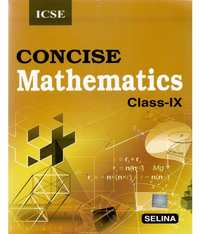## Chapter 9: Triangles [Congruency in Triangles]

Exercise 9 (A)Exercise 9 (B)
Exercise 9 (A) [Pages 122 - 123]

### Selina solutions for Concise Mathematics Class 9 ICSE Chapter 9 Triangles [Congruency in Triangles] Exercise 9 (A) [Pages 122 - 123]

Exercise 9 (A) | Q 1.1 | Page 122

If the following pair of the triangle is congruent? state the condition of congruency :
In Δ ABC and Δ DEF, AB = DE, BC = EF and ∠ B = ∠ E.

Exercise 9 (A) | Q 1.2 | Page 122

If the following pair of the triangle is congruent? state the condition of congruency :
In  ΔABC and ΔDEF,  ∠B = ∠E = 90o; AC = DF and BC = EF.

Exercise 9 (A) | Q 1.3 | Page 122

If the following pair of the triangle is congruent? state the condition of congruency:

In ΔABC and ΔQRP, AB = QR, ∠B = ∠R and ∠C = P.

Exercise 9 (A) | Q 1.4 | Page 122

If the following pair of the triangle is congruent? state the condition of congruency:

In ΔABC and ΔPQR, AB = PQ, AB = PQ, and BC = QR.

Exercise 9 (A) | Q 1.5 | Page 122

If the following pair of the triangle is congruent? state the condition of congruency:

In ΔADC and ΔPQR, BC = QR, ∠A = 90o, ∠C = ∠R = 40o and ∠Q = 50o.

Exercise 9 (A) | Q 2 | Page 122

The given figure shows a circle with center O. P is mid-point of chord AB.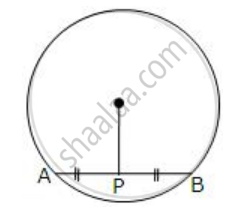Show that OP is perpendicular to AB.

Exercise 9 (A) | Q 3 | Page 122

The following figure shows a circle with center O.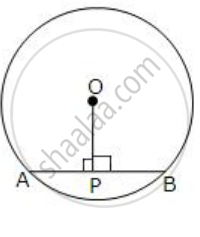If OP is perpendicular to AB, prove that AP = BP.

Exercise 9 (A) | Q 4 | Page 122

In a triangle ABC, D is mid-point of BC; AD is produced up to E so that DE = AD.
Prove that :
(i) ΔABD and ΔECD are congruent.
(ii) AB = CE.
(iii) AB is parallel to EC

Exercise 9 (A) | Q 5.1 | Page 122

A triangle ABC has ∠B = ∠C.
Prove that: The perpendiculars from the mid-point of BC to AB and AC are equal.

Exercise 9 (A) | Q 5.2 | Page 122

A triangle ABC has B = C.
Prove that: The perpendicu...lars from B and C to the opposite sides are equal.

Exercise 9 (A) | Q 6 | Page 122

The perpendicular bisectors of the sides of a triangle ABC meet at I.

Prove that: IA = IB = IC.

Exercise 9 (A) | Q 7 | Page 122

A line segment AB is bisected at point P and through point P another line segment PQ, which is perpendicular to AB, is drawn. Show that: QA = QB.

Exercise 9 (A) | Q 8 | Page 122

If AP bisects angle BAC and M is any point on AP, prove that the perpendiculars drawn from M to AB and AC are equal.

Exercise 9 (A) | Q 9 | Page 122

From the given diagram, in which ABCD is a parallelogram, ABL is a line segment and E is mid-point of BC.
Prove that:
(i) ΔDCE ≅ ΔLBE
(ii) AB = BL.
(iii) AL = 2DC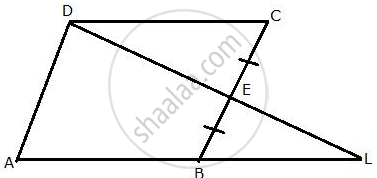Exercise 9 (A) | Q 10 | Page 122

In the given figure, AB = DB and Ac = DC.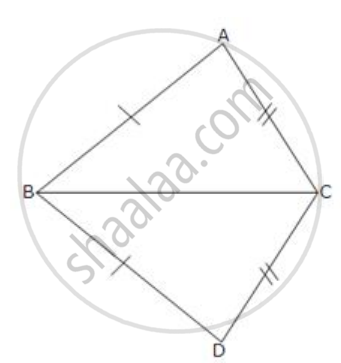If ∠ ABD = 58o,
∠ DBC = (2x - 4)o,
∠ ACB = y + 15o and
∠ DCB = 63o ; find the values of x and y.

Exercise 9 (A) | Q 11 | Page 122

In the given figure: AB//FD, AC//GE and BD = CE;
prove that:  (i) BG = DF       (ii) CF = EG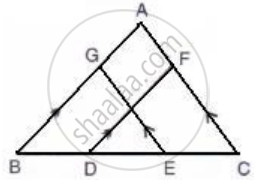Exercise 9 (A) | Q 12 | Page 122

In ∆ABC, AB = AC. Show that the altitude AD is median also.

Exercise 9 (A) | Q 13 | Page 122

In the following figure, BL = CM.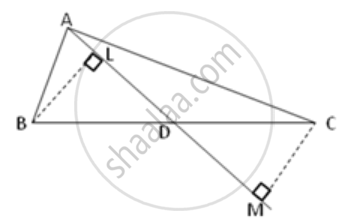Prove that AD is a median of triangle ABC.

Exercise 9 (A) | Q 14.1 | Page 123

In the following figure, AB = AC and AD is perpendicular to BC. BE bisects angle B and EF is perpendicular to AB.
Prove that:  BD = CD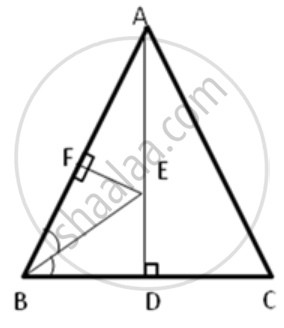Exercise 9 (A) | Q 14.2 | Page 123

In the following figure, AB = AC and AD is perpendicular to BC. BE bisects angle B and EF is perpendicular to AB.
Prove that :  ED = EFExercise 9 (A) | Q 15 | Page 123

Use the information in the given figure to prove :
(i) AB = FE
(ii) BD = CF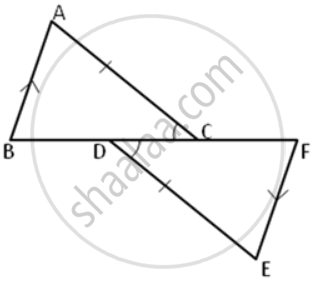Exercise 9 (B) [Pages 125 - 126]

### Selina solutions for Concise Mathematics Class 9 ICSE Chapter 9 Triangles [Congruency in Triangles] Exercise 9 (B) [Pages 125 - 126]

Exercise 9 (B) | Q 1 | Page 125

On the sides AB and AC of triangle ABC, equilateral triangle ABD and ACE are drawn.
Prove that:  (i) ∠CAD = ∠BAE
(ii) CD = BE

Exercise 9 (B) | Q 2.1 | Page 125

In the following diagram, ABCD is a square and APB is an equilateral triangle.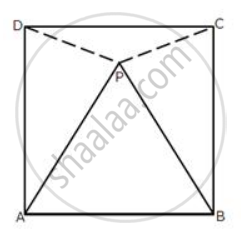(i) Prove that: ΔAPD≅ ΔBPC
(ii) Find the angles of ΔDPC.

Exercise 9 (B) | Q 2.2 | Page 125

In the following diagram, ABCD is a square and APB is an equilateral triangle.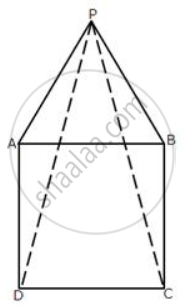(i) Prove that: ΔAPD ≅ ΔBPC
(ii) Find the angles of ΔDPC.

Exercise 9 (B) | Q 3 | Page 125

In the figure, given below, triangle ABC is right-angled at B. ABPQ and ACRS are squares.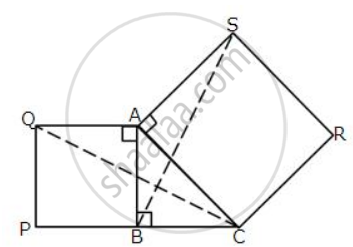Prove that:
(i) ΔACQ and ΔASB are congruent.
(ii) CQ = BS.

Exercise 9 (B) | Q 4 | Page 125

In a ΔABC, BD is the median to the side AC, BD is produced to E such that BD = DE.
Prove that: AE is parallel to BC.

Exercise 9 (B) | Q 5 | Page 125

In the adjoining figure, OX and RX are the bisectors of the angles Q and R respectively of the triangle PQR.
If XS ⊥ QR and XT ⊥  PQ ;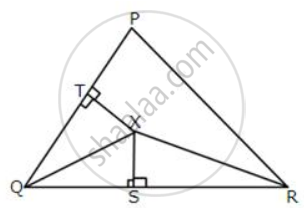prove that: (i) ΔXTQ ≅ ΔXSQ.
(ii) PX bisects angle P.

Exercise 9 (B) | Q 6 | Page 125

In the parallelogram ABCD, the angles A and C are obtuse. Points X and Y are taken on the diagonal BD such that the angles XAD and YCB are right angles.
Prove that: XA = YC.

Exercise 9 (B) | Q 7 | Page 125

ABCD is a parallelogram. The sides AB and AD are produced to E and F respectively, such produced to E and F respectively, such that AB = BE and AD = DF.
Prove that: ΔBEC ≅ ΔDCF.

Exercise 9 (B) | Q 8 | Page 125

In the following figures, the sides AB and BC and the median AD of triangle ABC are equal to the sides PQ and QR and median PS of the triangle PQR.
Prove that ΔABC and ΔPQR are congruent.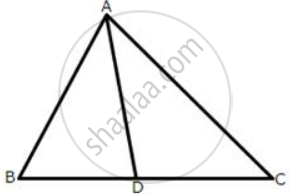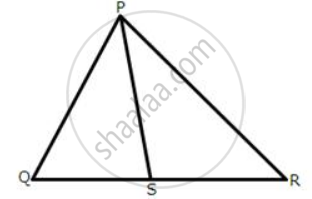Exercise 9 (B) | Q 9 | Page 126

In the following diagram, AP and BQ are equal and parallel to each other.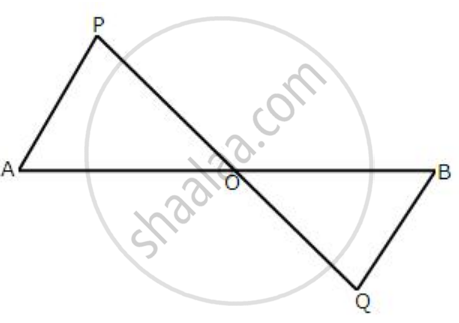Prove that:
(i) ΔAOP≅ ΔBOQ.
(ii) AB and PQ bisect each other.

Exercise 9 (B) | Q 10 | Page 126

In the following figure, OA = OC and AB = BC.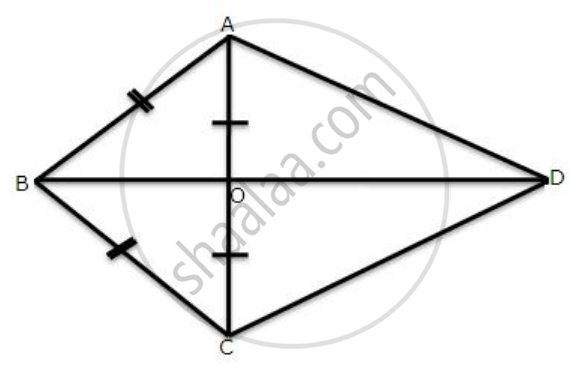Prove that:
(i) ∠AOB = 90o
(ii) ΔAOD ≅ ΔCOD

Exercise 9 (B) | Q 11.1 | Page 126

The following figure has shown a triangle ABC in which AB = AC. M is a point on AB and N is a point on AC such that BM = CN.
Prove that:  (i) AM = AN  (ii) ΔAMC ≅ ΔANB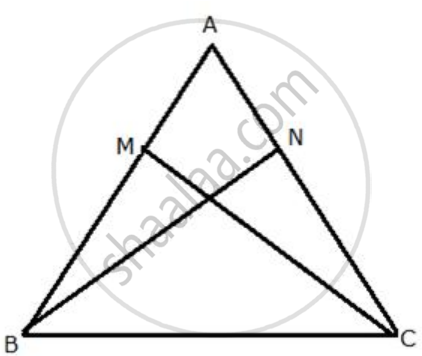Exercise 9 (B) | Q 11.2 | Page 126

The following figure has shown a triangle ABC in which AB = AC. M is a point on AB and N is a point on AC such that BM = CN.

Prove that:  (i) BN = CM (ii) ΔBMC≅ΔCNBExercise 9 (B) | Q 12 | Page 126

In a triangle, ABC, AB = BC, AD is perpendicular to side BC and CE is perpendicular to side AB.

Exercise 9 (B) | Q 13 | Page 126

PQRS is a parallelogram. L and M are points on PQ and SR respectively such that PL = MR.
Show that LM and QS bisect each other.

Exercise 9 (B) | Q 14 | Page 126

In the following figure, ABC is an equilateral triangle in which QP is parallel to AC. Side AC is produced up to point R so that CR = BP.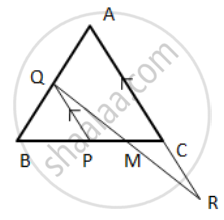Prove that QR bisects PC.
Hint: ( Show that ∆ QBP is equilateral
⇒ BP = PQ, but BP = CR
⇒ PQ = CR ⇒ ∆ QPM ≅ ∆ RCM ).

Exercise 9 (B) | Q 15 | Page 126

In the following figure, ∠A = ∠C and AB = BC.
Prove that ΔABD ≅ ΔCBE.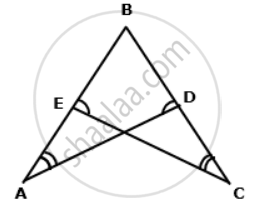Exercise 9 (B) | Q 16 | Page 126

AD and BC are equal perpendiculars to a line segment AB. If AD and BC are on different sides of AB prove that CD bisects AB.

Exercise 9 (B) | Q 17 | Page 126

In ΔABC, AB = AC and the bisectors of angles B and C intersect at point O.
Prove that : (i) BO = CO
(ii) AO bisects angle BAC.

Exercise 9 (B) | Q 18 | Page 126

In the following figure, AB = EF, BC = DE and ∠B = ∠E = 90°.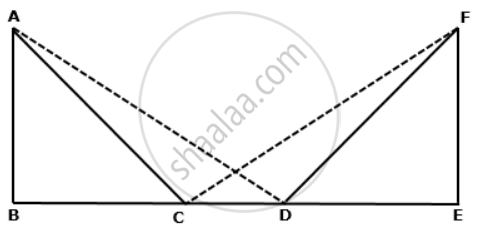Exercise 9 (B) | Q 19 | Page 126

A point O is taken inside a rhombus ABCD such that its distance from the vertices B and D are equal. Show that AOC is a straight line.

Exercise 9 (B) | Q 20 | Page 126

Prove that:
(ii) ∠DAB = ∠CBA

## Chapter 9: Triangles [Congruency in Triangles]

Exercise 9 (A)Exercise 9 (B)## Selina solutions for Concise Mathematics Class 9 ICSE chapter 9 - Triangles [Congruency in Triangles]

Selina solutions for Concise Mathematics Class 9 ICSE chapter 9 (Triangles [Congruency in Triangles]) include all questions with solution and detail explanation. This will clear students doubts about any question and improve application skills while preparing for board exams. The detailed, step-by-step solutions will help you understand the concepts better and clear your confusions, if any. Shaalaa.com has the CISCE Concise Mathematics Class 9 ICSE solutions in a manner that help students grasp basic concepts better and faster.

Further, we at Shaalaa.com provide such solutions so that students can prepare for written exams. Selina textbook solutions can be a core help for self-study and acts as a perfect self-help guidance for students.

Concepts covered in Concise Mathematics Class 9 ICSE chapter 9 Triangles [Congruency in Triangles] are Relation Between Sides and Angles of Triangle, Important Terms of Triangle, Congruence of Triangles, Criteria for Congruence of Triangles, Concept of Triangles - Sides, Angles, Vertices, Interior and Exterior of Triangle.

Using Selina Class 9 solutions Triangles [Congruency in Triangles] exercise by students are an easy way to prepare for the exams, as they involve solutions arranged chapter-wise also page wise. The questions involved in Selina Solutions are important questions that can be asked in the final exam. Maximum students of CISCE Class 9 prefer Selina Textbook Solutions to score more in exam.

Get the free view of chapter 9 Triangles [Congruency in Triangles] Class 9 extra questions for Concise Mathematics Class 9 ICSE and can use Shaalaa.com to keep it handy for your exam preparation